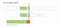I. Problem

Pattern Searching is very popular and important problem in computer science. We usually use it every day, like search a text in a browser, or in an editor. We can simply define the problem as:

There are some algorithms can resolve this problem. Knuth-Morris-Pratt algorithm (Aka KMP Algorithm) is one of these algorithms. From my point of view, KMP is not easy to understand at the first time looking into it, and even if we are not clear about it, we can easier to forget about this algorithm.

II. Naive Algorithm

Before looking into KMP. Firstly, we need to take a look at the naive algorithm. The KMP algorithm can consider as the improvement of naive algorithm.

In this algorithm, we will have a cursor which will compare two elements of pattern and txt,

We start at the first initialization state, which we put two string together. The cursor will start at the first index of both txt and pattern.

We compare two elements at the cursor. There are two possible case

• If they are equal: Then we move the cursor 1 step to the next element.
• If they are different: Then we shift the pattern 1 step and move back the cursor to the first index of pattern.

When the cursor moves over the pattern, that is mean we found the matching.

What is the complexity of this solution? In the worse case, we will shift lengthOf(txt) - lengthOf(pattern) steps. And in each shift, if we are unlucky, we need to move the cursor lengthOf(pattern) steps. Then the complexity is

where m is the length of pattern and n is the length of txt

III. KMP Algorithm

The KMP algorithm will improve the shifting part of the naive algorithm to prevent moving backward the cursor. So in every comparison, we will

• Move forward the cursor 1 step
• Or Shift the pattern more than 1 step

Until we found the matching. Then the complexity will be O(n). (We will prove it later in this article)

But how? How can we shift the pattern without move backward the cursor and still guarantee we can not miss the matching. Let see the situation when we in middle of comparison.

So, we are in the position which pattern[0 .. k-1] match to the txt. But the two elements from pattern and txt (which the cursor is pointing to) are different.

Because we want to keep the current position of the cursor, so we have to make sure that after we shift the pattern, every element from pattern to the element before the cursor still match with the part of txt. Like the picture below.

So now, the question is how we calculate the minimum steps of pattern shifting, to get the next matching like the above photo.

Focus on the above photo, do you find anything? Need more hint?Now, we put the previous state of the pattern and the new state of the pattern.

The second notice is the longer of the green part, the less of pattern shifting steps. And to calculate the green part we only need the pattern. Let assume lps[i] is the length of the longest string which:

• is prefix of pattern[0 .. i] include pattern[i]
• is suffix of pattern[0 .. i] include pattern[i]
• is not pattern[0 .. i]

After knowing the lps array (longest prefix suffix array). Let look back to the above picture, in the before state. After calculate and know that pattern[k] is not matching. We will shift the pattern to the new position which the cursor points to pattern[lps[k-1]] [Now lps[k-1] elements from pattern (of pattern) is match to txt].

With helper array lps, every comparison we will

Moving cursor plus shifting pattern will cost O(n).

Prove:

The cursor can move maximum n steps

The pattern can shift maximum (n-m) steps

=> Maximum steps is n + (n-m) = 2n -m

=> Big-O is O(n)

But what about the lps array? What is big-O of pre-calculation the lps array?

We will calculate lps by dynamic programming. Or simply we calculation lps[k] by lps[k-1], lps[k-2], … , lps

Assume that lpsString[i] is longest prefix suffix string of pattern[0 .. i] (include pattern[i])

We will know that

• => The string made from lpsString[k-1] + pattern[lps[k-1]] can not be of pattern[0 .. k].
• => Next possible expect: will be longest prefix suffix string of the one we used to expect: lpsString[k-1]. (= lpsString of lpsString[k-1])
• So we continue to compare pattern[k] with the element right after lpsString[lpsString[k-1]]. And so on we do the same until we get the match or the current longest prefix suffix string (which used to compare) is empty.

If you look at the way we calculate the lps array, we will see that: they are quite similar to the way we search pattern in txt. It is all about moving the cursor (cursor move step by step to calculate lps[i]) and shifting pattern (shifting to calculate the previous lps).
So the complexity is same: O(2 * m) = where m is the length of the pattern.

The code for building lps array by Kotlin

The code to find first occurrence position by KMP algorithm

Writing for remembering

More from Pi Vinci

Writing for remembering